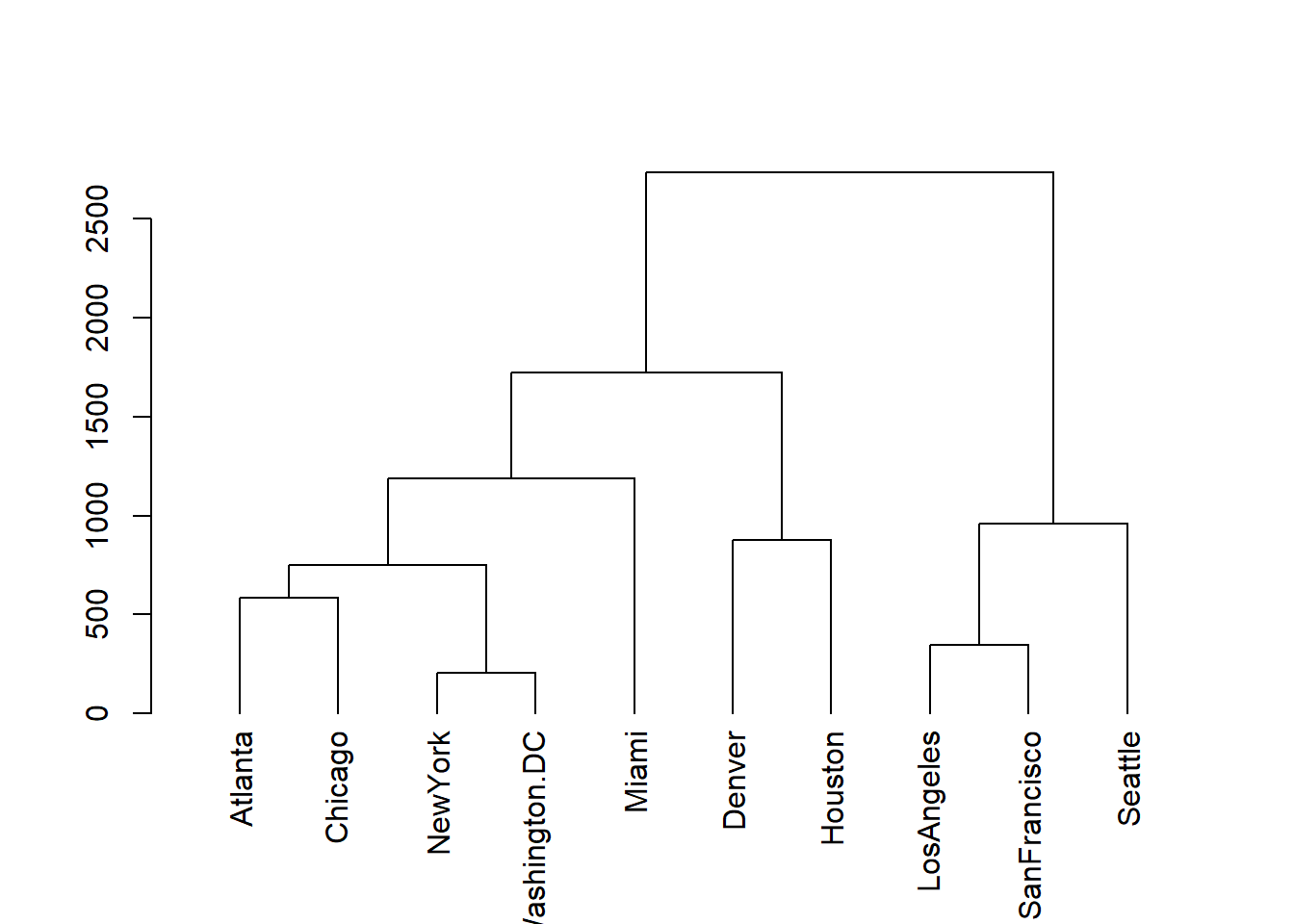## Description

R package mdendro enables the calculation of agglomerative hierarchical clustering (AHC), extending the standard functionalities in several ways:

• Native handling of both similarity and dissimilarity (distances) matrices.

• Calculation of pair-group dendrograms and variable-group multidendrograms .

• Implementation of the most common AHC methods in both weighted and unweighted forms: single linkage, complete linkage, average linkage (UPGMA and WPGMA), centroid (UPGMC and WPGMC), and Ward.

• Calculation of the cophenetic (or ultrametric) matrix.

• Calculation of five descriptors of the final dendrogram: cophenetic correlation coefficient, space distortion ratio, agglomerative coefficient, chaining coefficient, and tree balance.

• Plots of the descriptors for the parametric methods.

All this functionality is obtained with two functions: linkage, and descplot. Function linkage may be considered as a replacement for functions hclust (in package stats) and agnes (in package cluster). To enhance usability and interoperability, the linkage class includes several methods for plotting, summarizing information, and class conversion.

## Installation

There exist two main ways to install mdendro:

• Installation from CRAN (recommended method):

install.packages("mdendro")

RStudio has a menu entry (Tools $$\rightarrow$$ Install Packages) for this job.

• Installation from GitHub (you may need to install first devtools):

install.packages("devtools")
library(devtools)
install_github("sergio-gomez/mdendro")

Since mdendro includes C++ code, you may need to install first Rtools in Windows, or Xcode in MacOS.

## Tutorial

### Basics

Let us start by using the linkage function to calculate the complete linkage AHC of the UScitiesD dataset, a matrix of distances between a few US cities:

library(mdendro)
lnk <- linkage(UScitiesD, method = "complete")

Now we can plot the resulting dendrogram:

plot(lnk)The summary of this dendrogram is:

summary(lnk)
## Call:
##         type.prox = "distance",
##         digits = 0,
##         method = "complete",
##         group = "variable")
##
## Binary dendrogram: TRUE
##
## Descriptive measures:
##       cor       sdr        ac        cc        tb
## 0.8077859 1.0000000 0.7738478 0.3055556 0.9316262

In particular, you can recognize the calculated descriptors:

• cor: cophenetic correlation coefficient
• sdr: space distortion ratio
• ac: agglomerative coefficient
• cc: chaining coefficient
• tb: tree balance

It is possible to work with similarity data without having to convert them to distances, provided they are in range [0.0, 1.0]. A typical example would be a matrix of non-negative correlations:

sim <- as.dist(Harman23.cor\$cov)
lnk <- linkage(sim, type.prox = "sim")
plot(lnk, main = "Harman23")